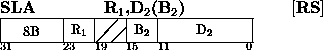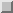Next: Shift Left Single Up: Insert Character Previous: Shift Left Double

### Shift Left SingleThe integer part of the first operand is shifted left the number of bits specified by the second-operand address. Bits 16-19 of the instruction are ignored.

The second-operand address is not used to address data ; ix low-ordered six bits indicate the number of bit positions to be shifted. The remainder of the address is ignored.

The sign of the first operand remains unchanged. All 31 integer bits of the operand participate in the left shift. Zeros are supplied to the vacated low-order register positions.

If a bit unlike the sign bit is shifted out of position 1 , an overflow occurs. The overflow causes a program interruption when the fixed-point overflow mask bit is one.

Resulting Condition Code:
0 ~ Result is zero
1 ~ Result is less than zero
2 ~ Result is greater than zero
3 ~ Overflow
Program Exceptions:
Fixed-Point Overflow

Programming Note
For numbers with an absolute value of less than 230 a left shift of one bit position is equivalent to multiplying the number by two.

Shift amounts from 31-63 cause the entire integer to be shifted out of the register. When the entire integer field for a positive number has been shifted out, the register contains a value of zero. For a negative number, the register contains a value of -231

See Extended Mnemonic Instructions on pageNext: Shift Left Single Up: Insert Character Previous: Shift Left Double

A.Daviel,TRIUMF